# New Qualitative Method to Investigate the Vibration Behavior of Orthotropic Composite Plates: Thickness Effect

New Qualitative Method to Investigate the Vibration Behavior of Orthotropic Composite Plates: Thickness Effect
Mechanical Engineering Department, Sciences of Technology Faculty, University Mentouri Constantine, Algeria

Corresponding Author Email:
samy11.hamada@gmail.com
Page:
141-147
|
DOI:
https://doi.org/10.18280/mmc_b.882-413
Received:
9 April 2019
|
Accepted:
5 December 2019
|
Published:
30 December 2019
| Citation

OPEN ACCESS

Abstract:

In this paper, we propose a quick and easy method to investigate the vibration behavior of orthotropic composite plates under the effect of thickness. In previous works, many investigations were carried out to challenge the effectiveness of this new method. To get insight about the level of accuracy of this method, the effect of boundary conditions, rigidity ratio and the effect of dimension ratio were discussed. The prediction of frequency responses using the qualitative method match very well with those obtained from finite element method. The first six frequency modes are obtained from the results achieved by the finite element method using ANSYS. The comparative and qualitative analysis of frequency responses allowed the justification of the position and the threshold of the respective modal frequencies of the plates in accordance with the results obtained from the numerical and analytical methods in use.

Keywords:

free vibration, composite, equivalent method, Rayleigh's method, finite element method.

1. Introduction

Nowadays the calculation of structures, irrespective of their complexities, is facilitated by the availability of software using very efficient finite element codes (FEC) of calculation. Although this type of software is sometimes very expensive, it is nonetheless often essential for the domains of leading-edge technology. Usually before their widespread distribution, those pieces of software are standardized and tested using comparative analyses with regard to the known results obtained from laboratory testing or using other calculation sources (finite elements, analytics).

During the investigation process of the mechanical behavior of structures, using a piece of software or a program for the calculation of structures, risks of calculation errors connected to the human factor can subsist at various levels of the analysis. These risks of errors can lead to costly and sometimes prejudicial expertise in relation to the good design of the structures.

In order to overcome this disadvantage, this work aims to minimize the risk of errors by providing an overall investigation strategy based on a new simple and fast qualitative method of expertise used in conjunction with the known results obtained according to the means of calculation based on an efficient finite element method (FEM) calculation code or other alternative methods. This analysis strategy is proposed in the context of the specific study of vibrating rectangular plates. However, it can be broadened to other types of structures based on adequate qualitative methods of estimation.

The use of orthotropic plates is becoming more and more common in several engineering fields such as aeronautical, aerospace, civil engineering and sports equipment. Hence the use of such structures requires an exact investigation to develop a precise and reliable design in the case where the physical properties of the material and the limit conditions are known.

From a technical point of view, the FEM provides a complete solution to the problem of evaluation of vibration modes and dynamic responses. On the other hand, when the main goal in the preliminary phase of design is proportioning (selection of geometric as well as mechanical properties of the material), we use simplified analytical models and approximate analytical methods in order to define a first impression of the vibration behavior from a general point of view.  This is also valid within the context of verification or expertise of calculation results such as those obtained by means of an efficient code of calculation (FEM).

Usually the numerical simulation using FEM software constitutes for some a supposedly exact reference to be used in comparative analyses. This standard procedure can sometimes cost precious time and require a highly qualified level of expertise.  In any case, the use of computer science through a piece of software always leaves the way open for a risk of error linked to human factor.  Consequently, it is useful in the first place to have an investigation strategy so as to achieve this double goal.  Firstly, one can calculate the intrinsic dynamic properties of the structure such as the modal frequencies of orthotropic plates, and define a first appreciation of the behavior of the plate as a whole.  Secondly, one can decrease the risk of errors of uneconomical calculation from the point of view of both cost and completion time.

In terms of quantitative investigation, numerous methods have been proposed in order to study the vibration of orthotropic plates; these methods are equally analytical and numerical. One can cite the articles gathered together by Leissa & Bert [1-9] discussing the dynamic behavior of composite and sandwich plates. Due to its high versatility and conceptual simplicity, the Rayleigh-Ritz method  is considered to be the reference for obtaining an approximate solution for the frequencies of a rectangular plate.

This method began with the article of Hearmon  who initiated the study of some particular cases. Among the works based on the Rayleigh-Ritz method, the contribution of Marongoni et al.  is used. This contribution is a combination of the Rayleigh-Ritz for upper bound and the decomposition method proposed by Bazely  for lower bound evaluation. In addition, for higher modes of modal frequencies, a special form of Rayleigh’s method is proposed by Biancolini . Thus, evaluation of higher frequencies is reduced to the calculation of the fundamental frequency of plates under the original plate.

However, in terms of qualitative vibration analysis, unfortunately no literary contributions were to be found. This is the reason why, to the best of our knowledge, this work is an original initiation that fills this inadequacy in order to provide engineers and researchers with a quick and simple method for the qualitative prediction and qualitative investigation of the vibration behavior of rectangular plates, taking into consideration all possible cases of modal sequences.

In this work, a simplified method is proposed to evaluate, from a qualitative point of view, the natural frequencies of an orthotropic plate based on an observation of the number of links existing or inherent to the plates and sub-plates activated by higher vibration modes. The principle of the analysis consists in counting the number of supports according to the boundary conditions of the plate or sub-plate, triggered by modal sequences during the free vibration of the rectangular plate.

As an example, for the obvious case of an isotropic rectangular plate undergoing two hypotheses cases respectively, simply supported plate (SSSS) and fully clumped plate (CCCC), it can be quickly verified that the latter case produces an upper threshold for the frequency of the fundamental mode compared to that of the simply supported plate. This obvious fact can be easily understood knowing that the proper value of the fundamental mode is directly proportional to the overall rigidity of the plate, which itself depends on the inherent boundary conditions.

If we limit ourselves to the hypothesis of the transverse vibration, we can consider the definition of a clumped support to be equivalent to two links preventing two degrees of freedom (vertical displacement and rotation). One can then notice, if we bear in mind the hypothesis which considers the small direction dominates the total vibratory behavior of the plate, that the number of connections of the clumped plate is (Plate CCCC → beam CC = 2C = 4S), Whereas, the number of connections of the simply supported plate is (Plate SSSS → beam SS = 2S).  It can therefore be seen that for the clumped plate, the number of connections is twice that of the simply supported plate and that its threshold frequency is necessarily higher.

This observation is less evident in the case of non-isotropic plates because, in addition to the effect of the boundary conditions, other factors influencing the overall stiffness of the plate are imposed. It is thus necessary to consider all possible cases of hypotheses that may limit the procedure of qualitative analysis. The main purpose of this work is to generalize the use of the qualitative method by overcoming all cases of hypotheses that may limit or obstruct the good judgment of the analysis.

Finally, it should be mentioned that the proposed method finds its relevance as a means of checking the coherence of the analysis results often presented in the form of a set of frequency curves expressed with respect to the numbers of vibration modes. Whatever the situation and the degree of complexity of the graphs cluttered with the comparison curves of the results, the qualitative method presented here allows a better visibility and a fast judgment on the coherence and the quality of the answers to the analysis.

The qualitative method is essentially based on the theory of long plates which behave like equivalent beams. The behavior, in this case, is dominated by the small direction of the rectangular plate. In this work, a quick comparative study concerning the threshold of both upper and lower rigidities and therefore, that of the lower and upper frequencies, is achieved by simple observation whilst counting the number of supporting links provided by the boundary conditions.

In previous works, in the case of isotropic plates, the effect of boundary conditions has been taken into account, the results obtained by the qualitative method were satisfying compared to those obtained through the finite element method. Beside the boundary conditions, we extended our work to investigate this new method in the case where several dimension ratios are introduced . The results have shown a good agreement.

By trying to further challenge the accuracy of the qualitative method, our work is extended to investigate and globalize this new method by introducing the study of orthotropic plates and the influence of different bending rigidity ratios . The accuracy of the procedure was tested in both cases where we have a dimension ratio (a/b>1) and (a/b<1), and the results maintain a good concordance with the results of the finite element method. In the present article, we envisage the influence of the thickness in the case of the hypothesis of thick plates.

2. Background Theory

The determination of frequencies, outside the simply supported case , presents difficulties in the integration of differential equations of dynamics motion of 4th order. That is why we need to use approximate methods.

An orthotropic material is characterized by the fact that its elastic mechanical properties have two symmetrical plans, thus only four independent elastic constants specifically E1, E2, G12, ν12 are considered. The coefficient ν12 can be determined using the equation:

$\frac{E_{1}}{E_{2}}=\frac{v_{12}}{v_{21}}$;         (1)

Using the hypotheses of Love-Kirchoff which neglect the effect of the shear forces and the rotational inertia, and introducing the parameters:

$D_{1}=\frac{E_{1} h^{3}}{12 \mu}, D_{2}=\frac{E_{2} h^{3}}{12 \mu}, D_{12}=\frac{G_{12} h^{3}}{12}$

$\mu=1-v_{12} v_{21}, 2 H=v_{21} D_{1}+v_{12} D_{2}+4 D_{12}$          (2)

with reference to figure 1 , the equation of the motion follows:

\begin{aligned} D_{1} \frac{\partial^{4} w}{\partial x^{4}}(x, y, t)+D_{1} & \frac{\partial^{4} w}{\partial y^{4}}(x, y, t)+2 H \frac{\partial^{4} w}{\partial^{2} \partial^{2}}(x, y, t) \\ &+\rho h \frac{\partial^{2} w}{\partial t^{2}}(x, y, t)=0 \end{aligned};          (3)

Considering a solution with general form:

$w=W(x, y)(A . \cos (\omega t)+B . \sin (\omega t))$;          (4)

And from former equations, it is possible to obtain an expression of two variables only:

$D_{1} \frac{\partial^{4} W}{\partial x^{4}}(x, y)+D_{1} \frac{\partial^{4} W}{\partial y^{4}}(x, y)+2 H \frac{\partial^{4} W}{\partial x^{2} \partial y^{2}}(x, y)$

$+\Lambda^{4} W=0$          (5)

## 1.pngFigure 1. Geometry of the model adopted in the paper

with: $\Lambda^{2}=\omega \sqrt{\rho h}$; (6)

Equation (5) must be solved to satisfy the following limit conditions:

M = 0, R = 0 for free side (F)

M = 0; W = 0 for simply supported side (S)

W = 0; $\partial W / \partial x(\text {or } \partial W / \partial y)=0$ for clamped side (C)

Table 1. Mechanical properties 

 Mechanical properties E1 (MPa) E2 (MPa) G12 (MPa) $\boldsymbol{v}_{\mathbf{1} 2}$ ρ (Kg/m3) Isotropic material 1E+10 1E+10 4.17E+09 0.2 7800 Orthotropic material 1E+10 5E+09 3.1E+09 0.2 7800

Table 2. Geometrical properties

 Model 1 Model 2 Model 3 Model 4 Model 5 Lx (m) 4 4 4 4 4 Ly (m) 2.667 2.667 2.667 2.667 2.667 Thickness (h) (m) 0.001 0.005 0.01 0.025 0.05
3. Discussion of Results

3.1 Finites elements method

The response according to finite element analysis is based on some models of rectangular meshing with (20X20), (40X40), (80X80) and (120X120). The case of (80X80) has been taken as a reference of comparison . The precision of investigation method is based on the study of a plate constitute of one isotropic material and one orthotropic material.

The calculation of the higher modal frequencies is executed considering the following main hypotheses: 

• the nodal lines are rectilinear and divide the plate in different parts forming rectangular plates;
• all the resultant parts vibrate at the same frequency;
• the modal shape of each part is the same that part would have if separated from the original plate and restrained with the proper conditions.

3.2 Dynamic investigation

The dynamic investigation is based on some evaluation criteria including dimension ratio, boundary conditions, vibration modes and material effect. According to those factors, analysis has permitted to understand some aspects of plate vibratory behavior.

The influence of the augmentation of the dimension ratio (LX/LY) indicates a parabolic augmentation. Long plates in one direction or another, and regardless of material reduces the contribution of rigidity in long sense which reduces vibration behavior to that of a beam supported on two elongated boards with boundary conditions of the same edges and the small sense. A qualitative and quantitative dynamic method have been proposed, based on the concept of long plates, which are dominated by the behavior of oriented beam according to the small sense of rectangular plate supposed infinite.

3.3 Influence of thickness and the boundary conditions on the behavior of free vibration of orthotropic rectangular plates

We have two orthotropic plates subjected to the limit conditions (SCSC) and (SSCC), we change the thickness (h[m]= 0.001, 0.005, 0.01, 0.025, 0.05), to verify the frequency curves and ensure their relatives proper positions progressively to their modal progressions.

We noticed an alternation of frequency factors gradually as evolution growing of modal number N1, N2, N3, N4, N5, N6. In order to justify and validate this behavior, qualitative analysis exploiting approximate method of Hearmon, which is useful for upper modes, is also used.

## 2.png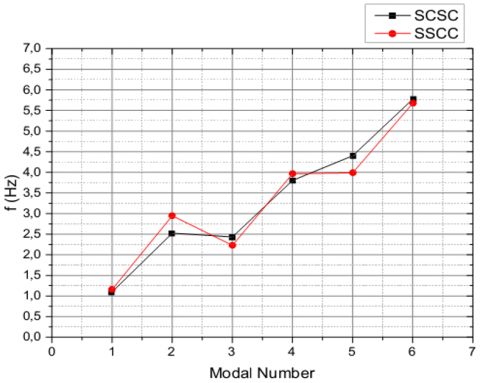Figure 2. Evolution of frequencies according to modal number and fixities conditions

Plate type SCSC: The vibration behavior still under the control of the small side along the (y) direction, we get a vibration contribution of a beam have both sides simply supports [SCSC⟶SS]. Figure 3 show how this beam is restrained.

Justification & Validating of ω11. If we focus on the plate (a/b), we can observe, basing on the theory of infinite plate, that the behavior should be dominated by the small side (Ly=b). Two types of contributions will be discussed:

## 3.pngFigure 3. Qualitative analysis (mode 1)

Plate type SSCC: We get a vibration contribution of a beam having both clamped and roller supports [SSCC⟶CS]. Figure 3 show how this beam is restrained.

Now let us compare the two cases:

Plate SCSC: Mode N1 (ω11) involve 2 Roller (2S)

Plate SSCC: Mode N1 (ω11) involve 1 Clamps + 1 Roller (C+S)

## 4.png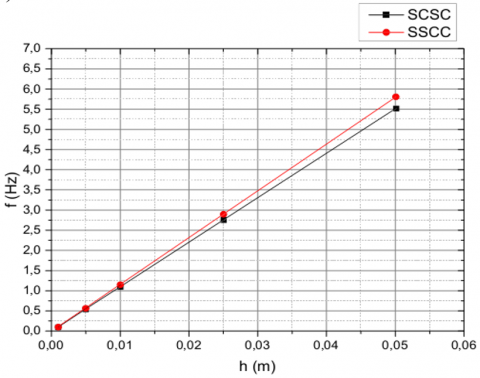Figure 4. effect of thickness on evolution of frequencies (mode 1)

Basing on qualitative analysis, we can say, since we have (2S) < (C+S) that we should have (ω11)SCSC < (ω11)SSCC because there is less restraint in plate SCSC than plate SSCC. This result is consistent with that given by the FEM analysis (figure2).

Now, by changing the thickness to see its influence on the behavior of the plate with the same restraint conditions, we can observe from the graphs (figure 4) that the order of the curves is respected, by means that we have always (ω11)SCSC<(ω11)SSCC, which mean that the change of the thickness doesn’t affect the behavior of the plate in the first mode N1.

Justification & Validating of ω12. Let us focus on the sub plate (a/b’) = [a/(b/2)], the behavior should be dominated by the small side (Ly = b). For the first plate (SCSC), we get vibration contribution of two types of the same beam SCSC [2(SS)]. Hence, for the second plate (SSCC), we also get vibration contribution of two beams, one having clamped and roller supports, and the other having pin support at one end and roller support at the other SSCC [(CS)+(SS)]. (Figure 5).

## 5.pngFigure 5. Qualitative analysis (mode 2)

Let's compare the two cases:

Plate SCSC: Mode N2 (ω12) involve 4 Roller (4S)

Plate SSCC: Mode N2 (ω12) involve 1 Clamps + 3 Roller (C+3S)

Basing on qualitative analysis, and since we have (4S)<(C+3S), we can say that we should have (ω12)SCSC<(ω12)SSCC. This result is consistent with that given by the FEM analysis (figure 2).

Now, by changing the thickness and by observing the graphs (figure 6), since the order of the curves is respected and the comparison (ω12)SCSC<(ω12)SSCC is available, we can deduce that the changes in thickness doesn't affect the behavior of the plate in the mode N2.

## 6.pngFigure 6. effect of thickness on evolution of frequencies (mode 2)

Justification & Validating of ω21. Qualitative analysis for mode (N3) for both plate cases (SCSC) and (SSCC) and focusing on the sub plate (a'/b)=[(a/2)/b], shows that the behavior should be dominated by the small side (Lx = a).

By comparing the two cases, we have:

Plate SCSC: Mode N3 (ω21) involve 2 Clamps + 2 Roller (2C+2S)

Plate SSCC: Mode N3 (ω21) involve 1 Clamps + 3 Roller (C+3S)

Since we have (C+3S) < (2C+2S), we should have (ω21)SSCC<(ω21)SCSC. This result is consistent with that given by the FEM analysis (figure 2).

Now, by changing the thickness and by observing the graphs (Figure 8), since the order of the curves is respected and the comparison (ω21)SCSC<(ω21)SSCC is available, we can deduce that the changes in thickness doesn’t affect the behavior of the plate in the mode N3.

## 7.png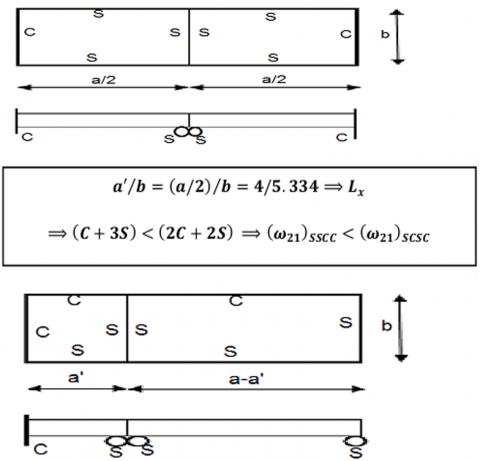Figure 7. Qualitative analysis (mode 3)

## 8.pngFigure 8. effect of thickness on evolution of frequencies (mode 3)

## 9.pngFigure 9. Qualitative analysis (mode 4)

Justification & Validating of ω22. Focusing on the sub plate (a'/b') = [(a/2)/(b/2)], shows that the behavior should be dominated by the small side (Ly = b). (Figure 9)

let's compare the two cases:

Plate SCSC: Mode N4 (ω22) involve 8 Roller (8S)

Plate SSCC: Mode N4 (ω22) involve 2 Clamps + 6 Roller (2C+6S)

Based on qualitative analysis and since we have (8S)<(2C+6S) we should have (ω22)SCSC < (ω22)SSCC because there is less restraint in plate (SCSC) than plate (SSCC). This result is confirmed by the FEM analysis (figure 2).

By changing the thickness and by observing the graphs (figure 10), since the order of the curves is respected and the comparison (ω22)SCSC < (ω22)SSCC is available, we can deduce that the changes in thickness doesn’t affect the behaviour of the plate in the mode N4.

## 10.pngFigure 10. Effect of thickness on evolution of frequencies (mode 4)

Justification & Validating of ω31. By focusing on the sub plate (a''/b) = [(a/3)/b] (Figure 11) and using qualitative analysis, we notice that the behavior is dominated by the side (Ly = b),

Thus, we have:

Plate SCSC: Mode N4 (ω31) involve 2 Clamps + 4 Roller (2C+4S)

Plate SSCC: Mode N4 (ω31) involve 3 Clamps + 3 Roller (3C+3S)

we should have (ω31)SSCC<(ω31)SCSC since we have (C+5S)<(2C+4S). This result is confirmed by the FEM analysis (figure 2).

## 11.pngFigure 11. Qualitative analysis (mode5)

## 12.png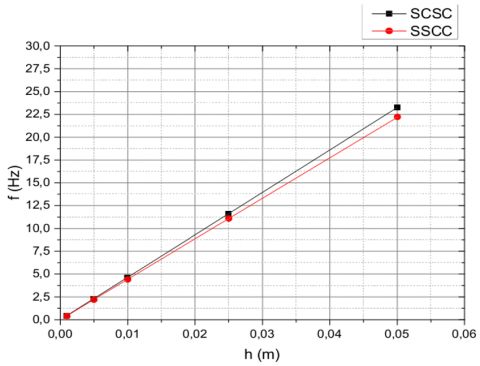Figure 12. effect of thickness on evolution of frequencies (mode 5)

Now, by changing the thickness and by observing the graphs (figure 12), since the order of the curves is respected and the comparison (ω31)SCSC<(ω31)SSCC is available, we can deduce that the changes in thickness doesn’t affect the behavior of the plate in the mode N5.

Justification & Validating of ω32. If we focus on the sub plate (a''/b’')=[(a/3)/(b/2)] (Figure 13) and using qualitative analysis, we notice that the behavior should be dominated by the small side (Lx=a), two types of contribution will be discussed:

## 13.pngFigure 13. Qualitative analysis (mode 6)

## 14.pngFigure 14. effect of thickness on evolution of frequencies (mode 6)

Plate SCSC: Mode N6 (ω32) involve 4 Clamps (4C) + 8 Roller (8S) (4C+8S)

Plate SSCC: Mode N6 (ω32) involve 2 Clamps + 10 Roller (2C+10S)

since we have (2C+10S) < (4C+10S) we should have (ω32)SSCC < (ω32)SCSC. This result is confirmed by the FEM analysis (figure 2).

Now, by observing the graphs (figure 14) and by changing the thickness, we notice that the order of curves is respected which mean that (ω32)SSCC<(ω32)SCSC and we can deduce that the changes in thickness doesn’t affect the behavior of the plate in the mode N6.

4. Conclusions & Recommendations

The main object of this work is to develop a simple qualitative method, polyvalent, efficient and precise in order to locate the frequency thresholds of rectangular plates.

A qualitative method is presented for the corroboration and assessment of vibration frequencies of rectangular plates. This rectangular plate is considered according to various parameters, in order to verify the credibility of the qualitative method, and at the same time, to determine the limits of its relative reliability, with regards to the results obtained by the usual calculations, which are often based on one of the qualitative numerical and or analytical methods documented in the literature.

This investigation is a complement to the previous works which help in testing the accuracy of the qualitative method by verifying and confirming the coherence of the frequency curves by studying their appropriate relative positions. The qualitative method of investigation used for the case of the isotropic rectangular plates has proven to be in perfect correlation with the frequency thresholds calculated in comparison with those anticipated qualitatively.

This method is now broadened and tested in the case of orthotropic plates. The investigation parameters are on top of the effect of the variation of the ratio of dimensions, of the ratio of flexional rigidity, and of the limit conditions. In this article, the effect of the variation of the orthotropic rectangular plate thickness is also considered. According to some of the general observations regarding the results discussed above, one can conclude that the qualitative method keeps its pertinence in estimating consistently the coherence of the quantitative results describing the behavior of this type of plates.

The accuracy of the procedure has been tested by comparing the natural frequencies obtained with the results of the FEM analysis of the same plate.

This study would be useful for concept engineers and research engineers by allowing them to reduce risks of errors linked to human factors and thus optimize the calculation time whilst reducing the realization costs.

The proposed approach is suitable for the calculation of preliminary designs as well as for the final qualitative control relating to the precision of the calculation results.

This method is not yet confirmed in the case of the square plates whose modal sequences evolve with complex forms by giving birth to non-linear modal lines of the rounded type. On the other hand, for future recommendations, the proposed qualitative method ought to undertake other investigations by taking into account other cases of rigidity ratio and of limit conditions and by considering the case of free boundaries as well as of elastic supports.

Nomenclature
 Lx length of the plate Ly width of the plate h thickness of the plate E1 young module in X axis E2 young module in Y axis G12 shear module $v_{12}$ Poisson coefficient ρ mass density w frequency FEC finite element codes FEM finite element method C Clumped edge S Simply supported edge CCCC totally clumped plate on all edges SSSS simplly supported plate on all edges
Appendix

Appendix 1. Results obtained from the FEM using ANSYS for plate SCSC

## a1.png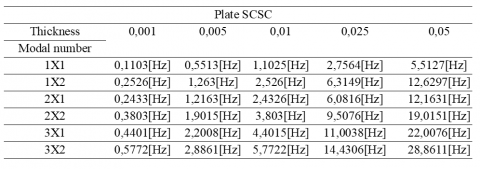Appendix 2. Results obtained from the FEM using ANSYS for plate SSCC

## a2.pngReferences

 Leissa, A.W. (1977). Recent Research in Plate Vibrations: Complicating Effects. Shock and Vibration Digest, 9(11): 21-35.

 Leissa, A.W. (1981). Plate vibration research 1976-1980: complicating effects. Shock and Vibration Digest, 13(10): 19-36. https://doi.org/10.1177/058310248101301004

 Leissa, A.W. (1987). Recent research in plate vibrations. 1981-1985 - Part II: complicating effects. Shock and Vibration Digest, 19(3): 10-24. https://doi.org/10.1177/058310248701900304

 Bert, C.W. (1976). Dynamics of composite and sandwich panels - Part I and II. Shock and Vibration Digest, 8(11): 15-24. https://doi.org/10.1177/058310247600801104

 Bert, C.W. (1979). Recent research in composite and sandwich plate dynamics. Shock and Vibration Digest, 11(10): 13-23. https://doi.org/10.1177/058310247901101003

 Bert, C.W. (1982). Research on dynamics of composite and sandwich plates. Shock and Vibration Digest, 14(10): 17-34. https://doi.org/10.1177/058310248201401006

 Bert, C.W. (1985). Research on dynamic behaviour of composite and sandwich plates - Part IV. Shock and Vibration Digest, 17(11): 3-25. https://doi.org/10.1177/058310248501701103

 Bert, C.W. (1991). Research on dynamic behaviour of composite and sandwich plates. V - Part I, Shock and Vibration Digest, 23(6): 3-14. https://doi.org/10.1177/058310249102300603

 Bert, C.W. (1991). Research on dynamic behaviour of composite and sandwich plates. V - Part II, Shock and Vibration Digest, 23(7): 9-21. https://doi.org/10.1177/058310249102300704

 Meirovitch, L. (1986). Elements of Vibration Analysis, McGraw-Hill, New York.

 Hearmon, R.F.S. (1946). The fundamental frequency of vibration of rectangular wood and plywood plates. Proceedings of the Physical Society of London, 58: 78-92. https://doi.org/10.1088/0959-5309/58/1/307

 Marangoni, R.D., Cook, L.M., Basavanhally, N. (1978). Upper and lower bounds to the natural frequencies of vibration of clamped rectangular orthotropic plates. International Journal of Solids and Structures, 14: 611-623. https://doi.org/10.1016/0020-7683(78)90001-X

 Bazely, N.W., Fox, D.W., Stadter, J.T. (1965). Upper and lower bounds for frequencies of rectangular clamped plates. Technical Memo. TG-626, Applied Physics Laboratory, The John Hopkins University, Baltimore, May.

 Biancolini, M.E., Butti, C., Reccia, L. (2005). Approximate solution for free vibration of thin orthotropic plates. Journal of sound and vibration 2005. https://doi.org/10.1016/j.jsv.2005.01.005

 Hamada, S., Benmansour, T. (2015). New qualitative method to investigate the vibration of composite rectangular plates under the effect of dimension and rigidities ratio of both perpendicular sides. International Journal of Mechanical and Production Engineering, 3(7): 26-31

 Hamada, S., Benmansour, T. (2014). Analysis of Complex Structures by Equivalent Methods: Application to Orthotropic Plates and the Effect of Rigidity Ratio. Proceeding of the International Symposium on Aircraft Materials ACMA 2014 Marrakech - Morocco April 23-26.

 Leissa, A.W. (1969). Vibration of Plates, NASA SP-160, Scientific and technical information Division of NASA, Washington, US, DC.

 Sekhri, A., Benmansour, T., Cheridi, H. (2011). Equivalents methods to investigate free vibration of isotropic and orthotropic thin rectangular plate with non-homogeneous supports. International Review of Mechanical Engineering, 5(7): 1196-1207.